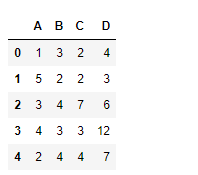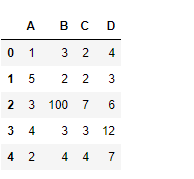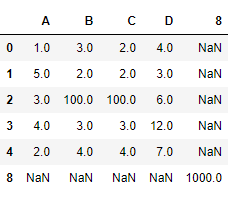# Python | Pandas dataframe.set_value()

• Last Updated : 24 Nov, 2018

Python is a great language for doing data analysis, primarily because of the fantastic ecosystem of data-centric python packages. Pandas is one of those packages and makes importing and analyzing data much easier.

Pandas` dataframe.set_value()` function put a single value at passed column and index. It takes the axis labels as input and a scalar value to be placed at the specified index in the dataframe. Alternative to this function is `.at[]` or `.iat[]`.

Syntax:DataFrame.set_value(index, col, value, takeable=False)
Parameters :
index : row label
col : column label
value : scalar value
takeable : interpret the index/col as indexers, default False

Return : frame : DataFrame If label pair is contained, will be reference to calling DataFrame, otherwise a new object

Example #1: Use `set_value()` function to set the value in the dataframe at a particular index.

 `# importing pandas as pd ` `import` `pandas as pd ` ` `  `# Creating the dataframe  ` `df ``=` `pd.DataFrame({``"A"``:[``1``, ``5``, ``3``, ``4``, ``2``], ` `                   ``"B"``:[``3``, ``2``, ``4``, ``3``, ``4``],  ` `                   ``"C"``:[``2``, ``2``, ``7``, ``3``, ``4``], ` `                   ``"D"``:[``4``, ``3``, ``6``, ``12``, ``7``]}) ` ` `  `# Print the dataframe ` `df `Lets use the `dataframe.set_value()` function to set value of a particular index.

 `# set value of a cell which has index label "2" and column label "B" ` `df.set_value(``2``, ``'B'``, ``100``) `

Output :Example #2: Use `set_value()` function to set value of a non-existent index and column in the dataframe.

 `# importing pandas as pd ` `import` `pandas as pd ` ` `  `# Creating the dataframe  ` `df ``=` `pd.DataFrame({``"A"``:[``1``, ``5``, ``3``, ``4``, ``2``], ` `                   ``"B"``:[``3``, ``2``, ``4``, ``3``, ``4``],  ` `                   ``"C"``:[``2``, ``2``, ``7``, ``3``, ``4``],  ` `                   ``"D"``:[``4``, ``3``, ``6``, ``12``, ``7``]}) ` ` `  `# Print the dataframe ` `df `Lets use the `dataframe.set_value()` function to set value of a particular index.

 `# set value of a cell which has index label "8" and column label "8" ` `df.set_value(``8``, ``8``, ``1000``) `

Output :Notice, for the non-existent row and column in the dataframe, a new row and column has been inserted.

My Personal Notes arrow_drop_up
Recommended Articles
Page :